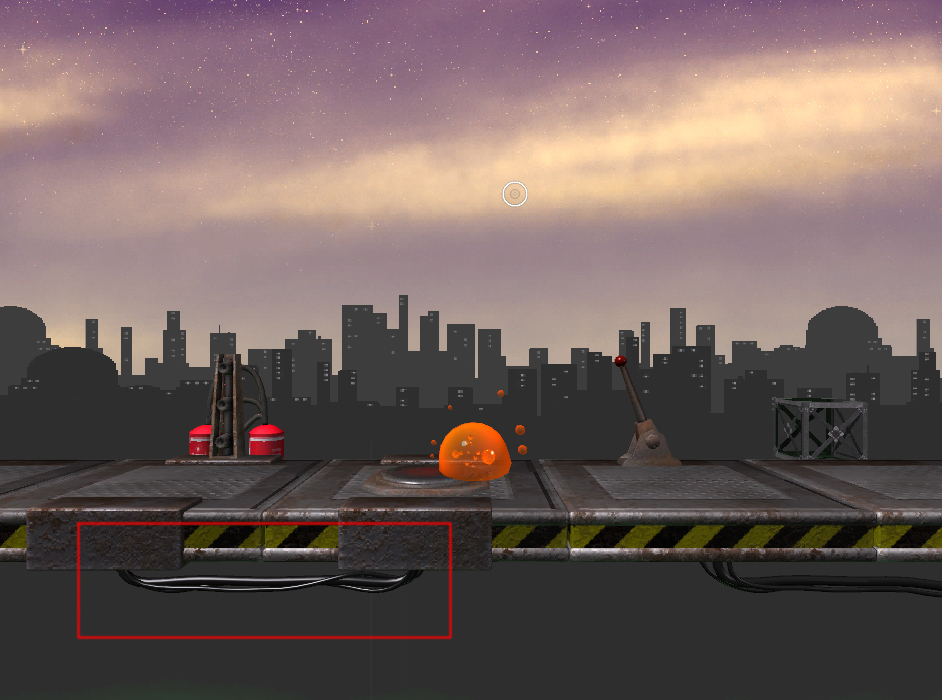# Procedural wires/cables/tubes

I am currently building a little puzzle platformer an currently I try to create a method to tell the player what button/lever control which mechanism, without outright telling them. I think the best way would be wires that connect the appropriate tiles. I created some wire models, but placing them is a bit tedious and I would have to create additional models for new situations, like wires going up or behind the platforms.

I thought about writing a script that creates wires between two objects, not necessarily as elaborate as the models I have now, but more user-friendly.

I think the separate steps would be:

• Create a master curve between two objects (probably cosh(x))
• Create one/multiple slave curves that follow the master spline, with added twists and turns
• Create vertices along the slave splines and connect them with polygons

While I think I can manage the first (although probably without being able to display the curve to test if it works), I have no idea how to start on the other two.

Image to show how it look right now:Ater failing to create meshes on runtime, I decided to use the LineRenderer instead. I am quite happy with it, although it seems to handle materials differently than the MeshRenderer, but it is just so much easier to use.
Anyways, here is the working code. Not sure if I want to work a bit more on it, or call it good enough…

``````using UnityEngine;
using System.Collections;

public class Spliner : MonoBehaviour {

public GameObject next;
public float gravity;
public int steps;

public int subSplines;
public float frequency;
public float amplitude;

public Material material;

private Vector3 curPosition;
private Vector3 nextPosition;
private Vector3[] mainPointArr = new Vector3;

private Vector3[,] subPointArr = new Vector3[0,0];

private GameObject[] children;

void Start() {

if(next) {
nextPosition = next.transform.position;
curPosition = transform.position;

if(steps < 2) steps = 2;

mainPointArr = new Vector3[steps + 1];

for(int i = 0; i <= steps; i++) {
mainPointArr *= DrawCosH(i);*
``````

}

if(subSplines < 1) subSplines = 1;

subPointArr = new Vector3[subSplines, steps];

for(int i = 0; i < subSplines; i++) {
DrawSubSpline(i);
}

children = new GameObject[subSplines];

for(int i = 0; i < subSplines; i++) {
children = new GameObject(“Child0” + i);
children*.transform.parent = transform;*
temp.SetVertexCount(steps);
temp.material = material;
for(int j = 0; j < steps; j++) {
temp.SetPosition(j, subPointArr[i, j]);
}
}
}
}

public Vector3 DrawCosH(int i) {
Vector3 direction = nextPosition - curPosition;
int locStep = steps - 1;

return curPosition + direction/locStep * i + new Vector3(0, gravity * (CosH((1.0f * i / locStep) - 0.5f) - CosH(0.5f)), 0);
}

float CosH(float t) {
return (Mathf.Exp(t) + Mathf.Exp(-t))/2;
}

void DrawSubSpline(int i) {
float locAmp = amplitude * Random.Range(0.5f, 1.2f) / 10;
float locFreq = frequency * Random.Range(0.8f, 1.2f);
float offset = Random.Range(0.0f, 1.0f);
int cw = (Random.Range(0.0f, 1.0f) > 0.5 ? -1 : 1);

for(int j = 0; j < steps; j++) {
Vector3 direction = mainPointArr[j + 1] - mainPointArr[j];
Quaternion rot = Quaternion.LookRotation(direction);

subPointArr[i, j] = mainPointArr[j] + rot * (Vector3.up * Mathf.Sin(1.0f * j * locFreq / steps + offset) + Vector3.right * cw * Mathf.Cos(1.0f * j * locFreq / steps + offset)) * locAmp;
}
}
}
I’ll keep this question open, in case someone views that as an invitation to borrow me his brain…

Hi man, this looks like a really good script there, one which i desperatly need, however i have no clue how to use itI’m kinda new to programming, and I use Java, so…